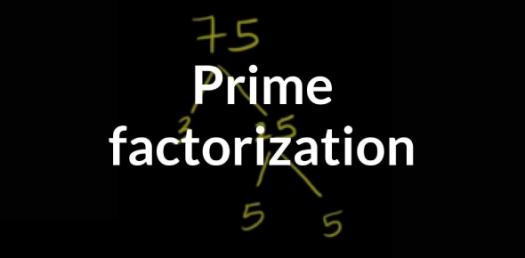# 5th Grade Math Quiz On Prime Factorization! Trivia Questions

6 Questions | Attempts: 3067SettingsWelcome to this 5th Grade Math Quiz on Prime Factorization! Factorization is the act of breaking down a number into a product of its factors and in this quiz, you will get some much-needed practice when it comes to these types of math problems. Do give it a shot and see how much more practice you may need.

• 1.
Is 3 a factor of 138?
• A.

Yes

• B.

No

• 2.
Is 2439 divisible by 9?
• A.

Yes

• B.

No

• 3.
Is 2442 divisible by 6?
• A.

Yes

• B.

No

• 4.
What is the prime factorization of 48?
• A.

2 x 2 X 2 x 2 x 3

• B.

2 x 2 x 2 x 3

• C.

2 x 3 x 4

• D.

2 x 12 x 2

• 5.
Find the prime factors of 520?
• A.

2 x 3 x 4 x 5

• B.

2 x 2 x 2 X 5 x 13

• C.

2 x 5 x 52

• 6.
What is the prime factorization of 603
• A.

2 x 7 x 43

• B.

3 x 3 x 67

• C.

3 x 201

## Related TopicsBack to top
×

Wait!
Here's an interesting quiz for you.